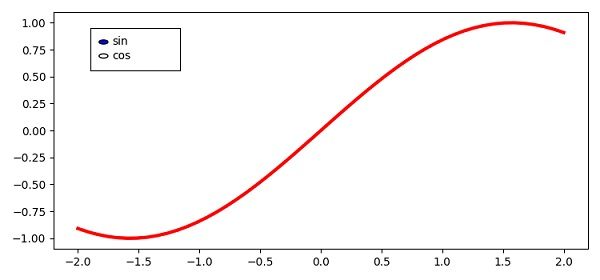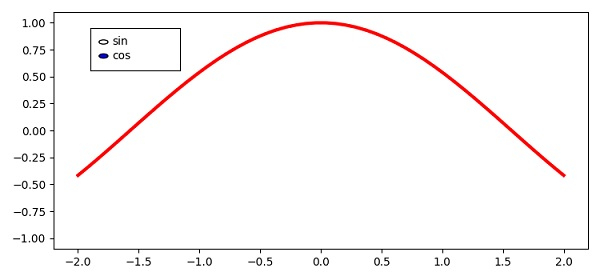# How to change the curve using radiobuttons in Matplotlib?

To change the color of a line using radiobuttons, we can take the following steps −

• Create x, sin and cos data points using numpy.

• Adjust the figure size and padding between and around the subplots.

• Create a figure and a set of subplots using the subplots() method.

• Plot curve with x and y data points using the plot() method.

• Add an axes to the current figure and make it the current axes, using the axes() method.

• To change the curve with radionbutton, we can use the change_curve() method that can be passed in on_clicked() method.

• To display the figure, use the show() method.

## Example

import numpy as np
from matplotlib import pyplot as plt

plt.rcParams["figure.figsize"] = [7.50, 3.50]
plt.rcParams["figure.autolayout"] = True

x = np.linspace(-2, 2, 50)
sin = np.sin(x)
cos = np.cos(x)
fig, ax = plt.subplots()
l, = ax.plot(x, sin, lw=3, color='red')
rb_axis = plt.axes([0.15, 0.75, 0.15, 0.15])

def change_curve(c_label):
d = {'sin': sin, 'cos': cos}
data = d[c_label]
l.set_ydata(data)
plt.draw()

plt.show()